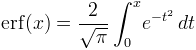Computes the error function.

Namespace:  Meta.Numerics.Functions
Assembly:  Meta.Numerics (in Meta.Numerics.dll) Version: 4.1.4Syntax
```public static double Erf(
double x
)```

#### Parameters

x
Type: SystemDouble
The argument.

#### Return Value

Type: Double
The value of erf(x).Remarks

The error function can be defined as a Gaussian integral.The area under a bell curve (NormalDistribution) within ∓z standard deviations of the mean is given by erf(z/√2).

For large values of x, erf(x) ≈ 1 to within floating-point accuracy. To obtain accurate values of erfc(x) = 1 - erf(x) in this range, use the Erfc(Double) function.

The inverse of the error function is available as InverseErf(Double).

Values of the error function for complex arguments can be obtained using Erf(Complex) method, or using the equivalent but re-parameterized Faddeeva(Complex) function.See Also